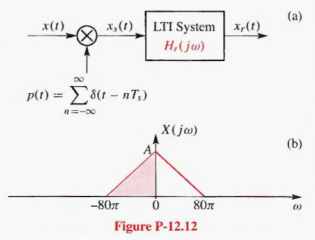# The derivation of the sampling theorem involves the operations of impulse train sampling and… 1 answer below »

The derivation of the sampling theorem involves the operations of impulse train sampling and reconstruction as shown in Fig. P- 12.12(a).

(a) For the input with Fourier transform depicted in Fig. P-12.12(b). use the sampling theorem to choose the sampling rate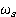= 2π/ TS, so that x1(t) = x(t) when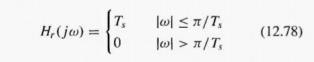Plot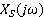for the value of= 2π/Ts, that is equal to the Nyquist rates

(b) If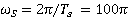in the above system and X(jω) is as depicted above. plot the Fourier transform X,(jω) and show that aliasing occurs. There will be an infinite number of shifted copies of X (jω). so indicate the periodic pattern as a function of ω.

(c) For the conditions of part (b), determine and sketch the Fourier transform of the output X,(jω) if the frequency response of the LTI system is given by (12.78).